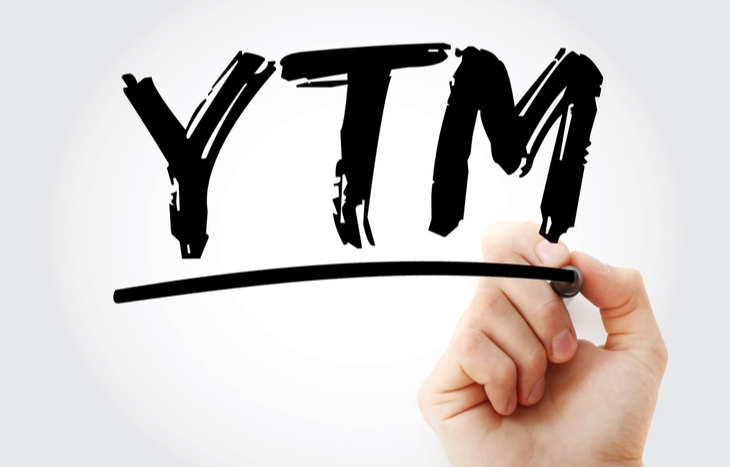One of the most popular measures of bond yield is yield to maturity (YTM). Also called book yield or redemption yield, it’s the estimated rate of return an investor can expect from a bond when held until maturity. Because it accounts for the time value of money, it’s often seen as the most accurate measure of yield for those willing to hold their bonds.

Yield to maturity is a useful comparison tool when it comes to evaluating potential bond investments. Investors can look ahead at anticipated returns over differing maturities and coupon rates to better-understand the best option for their investment time horizon.

Here’s a look at yield to maturity in the context of bond investments and how it stacks up as a measure of return against other metrics.## How to Calculate Yield to Maturity

Yield to maturity is a complex calculation because it involves forward-looking, compounding values. Most investors will use a bond maturity calculator to determine the YTM; however, there is a formula that can express the yield:

YTM = (Face Value / Current Price)(1/Time to Maturity) – 1

Manually calculating YTM can be difficult because investors need to consider if the face value is at par, discounted or at a premium. The formula also assumes that all coupon payments are reinvested at the same rate as the bond’s current yield. There can be some trial and error in determining what interest rate comes out closest to the bond price. Often, YTM is a calculation best left to a pre-programmed calculator.

## Bond YTM vs. Coupon Rate

One important fact to remember is that a bond’s yield to maturity is not the same as its coupon rate. The YTM is the estimated annualized rate of return on a bond held to maturity. Coupon rate is simply the bond’s stated interest rate. While different, there’s an important correlation between the two:

• If the coupon rate is higher than the bond’s YTM, it’s at a premium.
• When the YTM is higher than the bond’s coupon rate, it’s discounted.
• If the YTM and the coupon rate are relatively similar, the bond is fairly valued.

Because YTM is a representation of present value, it’s often used as a more accurate measure of bond value, as opposed to the given coupon rate. And, because bonds can trade for a premium or a discount, YTM offers a more accurate look at the bond’s true value, as opposed to simply comparing coupon rates.

## Yield to Maturity vs. Current Yield

Investors comparing bonds will often stack up current yield vs. YTM as a way of comparing bond values. It’s useful for understanding the impact of investing time horizon.

• Current yield is the interest it pays annually, divided by the bond’s current price. It approximates what a bond holder might earn over a year; however, because bond values fluctuate, it’s not a guarantee. If an investor buys a five-year \$1,000 bond with a \$50 coupon, its current yield is 5%. Selling that bond after two years for \$950 means the return was only \$50.
• Yield to maturity helps investors see the anticipated return on the bond if held to maturity. Using the same example, the investor with the five-year \$1,000 bond at a 5% coupon might see a yield to maturity of 6.2%, or a \$310 total return when held for the full five years.

The big difference here is that YTM factors in the present values of the future payments from a bond. Current yield doesn’t account for the time value of money, which can give an incomplete measure of the bond’s yield.

## YTM vs. Other Types of Yield

Because yield to maturity is a best-case representation of a bond’s yield over its full life, it’s often used as a benchmark. Here’s a look at other measures of yield it’s compared to:

• Yield to Worst (YTW). Lowest potential return available on a bond at risk of default. This type of yield measures the total yield on a bond over a short time period and is usually compared to YTM to show best and worst-case scenarios.
• Yield to Call (YTC). The measure of the bond’s yield at the time of its call date. It’s similar to YTW in that it examines the yield of a bond before its latest maturity date. It’s also stacked up against YTM and YTW to gauge value.

## Drawbacks When Examining Yield to Maturity

Yield to maturity is an assumptions-based formula, which means it’s prone to error, volatility and other unpredicted situations. For example, the bond holder may not reinvest all coupon payments. Or, the bond issuer may call the bond early. Moreover, the formula doesn’t account for taxes and sales costs that come with the bond. While it does a great job of contextualizing anticipated returns, YTM is more of an evaluation tool than anything else.

## YTM is the Internal Rate of Return for Bonds

For those who invest in securities, yield to maturity might sound more familiar as a different moniker: internal rate of return. This metric helps investors and analysts estimate the future profitability of an investment based on its expected annual growth rate. It’s also akin to net present value.

Regardless of how investors identify with it, YTM is an important metric used to discern the future value of a bond based on present variables. This information can give investors everything they need to make an informed, confident decision when selecting a bond that fits with their investment time horizon.